Function Repository Resource:

Get a numerically sorted list of abscissa-weight pairs for Clenshaw-Curtis quadrature

Contributed by: Jan Mangaldan
 ResourceFunction["ClenshawCurtisQuadratureWeights"][n,{a,b}] gives a list of the n pairs {xi,wi} of the n-point Clenshaw-Curtis formula for quadrature on the interval a to b, where wi is the weight of the abscissa xi. ResourceFunction["ClenshawCurtisQuadratureWeights"][n,{a,b},prec] uses the working precision prec.

## Details and Options

Clenshaw-Curtis approximates the value of an integral as a linear combination of values of the integrand evaluated at abscissas xi: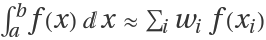.
Clenshaw-Curtis quadrature uses extrema of the Chebyshev polynomial ChebyshevT, augmented with ±1 and rescaled to the interval (a,b), as the abscissa values.
The precision argument acts similarly to the WorkingPrecision option used in many Wolfram Language numeric functions; it is not at all like the PrecisionGoal option. If the n argument is too small to allow for a high-precision result, extra precision in a result will not be meaningful.

## Examples

### Basic Examples (2)

The abscissas and weights for a 10-point Clenshaw-Curtis quadrature on a given interval:

 In:=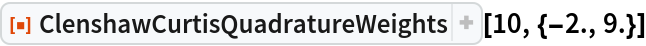Out=Use the specified precision:

 In:=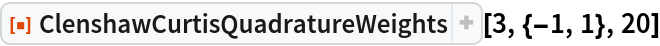Out=### Applications (4)

Use Clenshaw-Curtis quadrature to approximate the area under a curve:

 In:=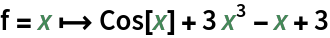Out=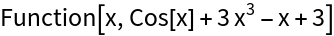Plot the curve over a given interval:

 In:=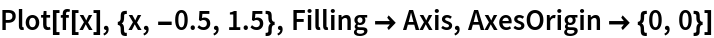Out=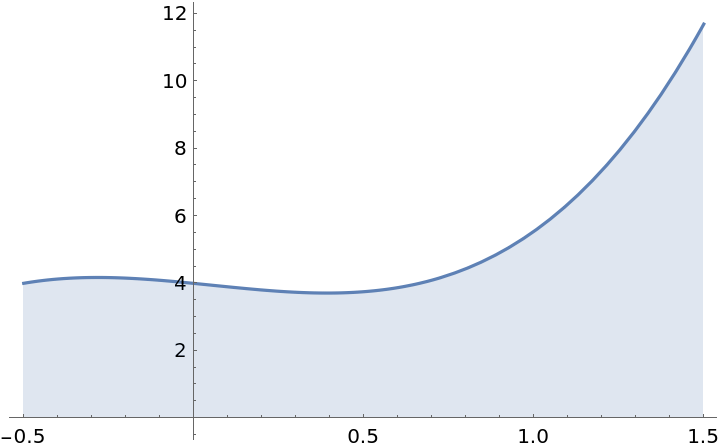Approximate the area under the curve using n-point Clenshaw-Curtis quadrature:

 In:=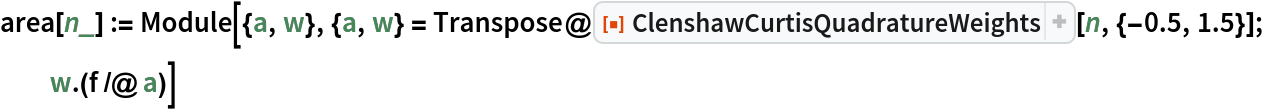In:=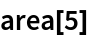Out=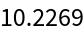Compare to the output of NIntegrate:

 In:=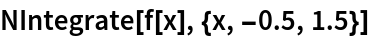Out=In:=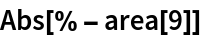Out=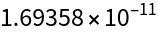### Properties and Relations (1)

The abscissas of n-point Clenshaw-Curtis quadrature are the extrema of the Chebyshev polynomial Tn-1(x), along with the interval extrema ±1:

 In:=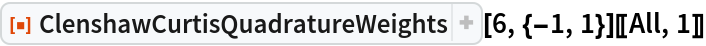Out=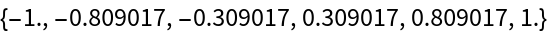In:=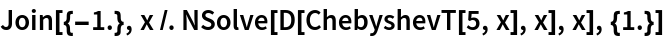Out=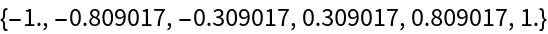## Version History

• 1.0.0 – 04 January 2021